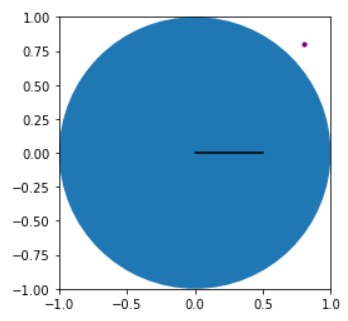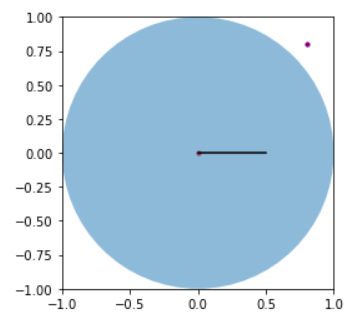hi,

# import matplotlib.pyplot as plt import numpy as np figure, axes = plt.subplots() draw_circle = plt.Circle( (0,0), 1, fill= True) axes.plot(np.linspace(0,0.5,50),np.zeros(50),color=“black”) axes.add_artist(draw_circle) axes.scatter(0,0,s=10,color=“red”) axes.scatter(0.8,0.8,s=10,color=“purple”) axes.set_aspect(1) axes.set_xlim(left=-1,right=1) axes.set_ylim(top=1,bottom=-1) plt.show()

Without setting alpha =0.5,centre is not visible, but the line is visibleAfter setting alpha = 0.5, the centre is visible.hi

Thanks so much for replying. The version of matplotlib I am using is 3.4.3.

Thanks.
Sam

Why are you not using 3.5.2? Using the latest version is suggested for you.

hi Cindy,

Thanks. Updated the matplotlib version. Then the dot come out.

Thanks,
Sam